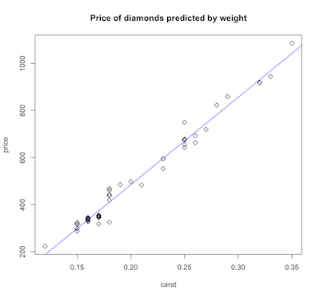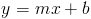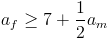## Saturday, August 21, 2010

### Using R for Introductory Statistics, Chapter 3.4

...a continuing journey through Using R for Introductory Statistics, by John Verzani.

#### Simple linear regression

Linear regression is a kooky term for fitting a line to some data. This odd bit of terminology can be blamed on Sir Francis Galton, a prolific victorian scientist and traveler who saw it as related to his concept of regression toward the mean. Calling it a linear model is a little more straight-forward, and linear modeling through the lm function is bread-and-butter to R.

For example, let's look at the data set diamonds to see if there's a linear relationship between weight and cost of diamonds.```f = price ~ carat
plot(f, data=diamond, pch=5,
main="Price of diamonds predicted by weight")
res = lm(f, data=diamond)
abline(res, col='blue')
```

We start by creating the formula f using the strange looking tilde operator. That tells the R interpreter that we're defining a symbolic formula, rather than an expression to be evaluated immediately. So, our definition of formula f says, "price is a function of carat". In the plot statement, the formula is evaluated in the context given by data=diamond, so that the variables in our formula have values. That gives us the scatter plot. Now let's fit a line using lm, context again given by data=diamond, and render the resulting object as a line using abline. Looks spiffy, but what just happened?

The equation of a line that we learned in high school is:Minimizing squared error over our sample gives us estimates of the slope and intercept. The book presents this without derivation, which is a shame.

Maybe later, I'll get brave an try to insert a derivation here.

#### Examples

There's a popular linear model that applies to dating, which goes like this: It's OK for a man to date a younger woman if her age is at least half the man's age plus seven. In other words, this:Apparently, I should be dating a 27 year old. Let me go ask my wife if that's OK. In the meantime, let's see how our rule compares to results of a survey asking the proper cutoff for dating for various ages.

```plot(jitter(too.young\$Male), jitter(too.young\$Female),
main="Appropriate ages for dating",
xlab="Male age", ylab="Female age")
abline(7,1/2, col='red')
res <- lm(Female ~ Male, data=too.young)
abline(res, col='blue', lty=2)
legend(15,45, legend=c("half plus 7 rule",
"Estimated from survey data"),
col=c('red', 'blue'), lty=c(1,2))
```

That's a nice correspondence. On second thought, this is statistical proof that my daughter is not allowed to leave the house 'til she's 30.

Somehow related to that is the data set Animals, comparing weights of body and brain for several animals. The basic scatterplot not revealing much, we put the data on a log scale and find that it looks much better. As near as I can tell, the I or AsIs function does something like the opposite of the tilde operator. It tells the interpreter to go ahead and evaluate the enclosed expression. The general gist is to transform our data to log scale then apply linear modeling.

```f = I(log(brain)) ~ I(log(body))
plot(f, data=Animals,
main="Animals: brains vs. bodies",
xlab="log body weight", ylab="log brain weight")
res = lm(f, data=Animals)
abline(res, col='brown')
```Now the problem is, the line doesn't seem to fit very well. Those three outliers on the right edge have high body weights but less than expected going on upstairs. That seems to unduly influence the linear model away from the main trend. R contains some alternative algorithms for fitting a line to data. The function lqs is more resistant to outliers, like the large but pea-brained creatures in this example.

```res.lqs = lqs(f, data=Animals)
abline(res.lqs, col='green', lty=2)
```That's better. Finally, you might use identify to solve the mystery of the knuckleheaded beasts.

```with(Animals, identify(log(body), log(brain), n=3, labels=rownames(Animals)))
```

Problem 3.31 is about replicate measurements, which might be a good idea where measurement error, noisy data, or other random variation is present. We follow the by now familiar procedure of defining our formula, doing a scatterplot, building our linear model, and finally plotting it over the scatterplot.We are then asked to look at the variance of measurements at each particular voltage. To do that, we'll first split our data.frame up by voltage. The result is a list of vectors, one per voltage level.

```breakdown.by.voltage = split(breakdown\$time, breakdown\$voltage)
str(breakdown.by.voltage)
List of 7
\$ 26: num [1:3] 5.8 1580 2323
\$ 28: num [1:5] 69 108 110 426 1067
\$ 30: num [1:11] 7.7 17 20 21 22 43 47 139 144 175 ...
\$ 32: num [1:15] 0.27 0.4 0.69 0.79 2.75 3.9 9.8 14 16 27 ...
\$ 34: num [1:19] 0.19 0.78 0.96 1.31 2.78 3.16 4.15 4.67 4.85 6.5 ...
\$ 36: num [1:15] 0.35 0.59 0.96 0.99 1.69 1.97 2.07 2.58 2.71 2.9 ...
\$ 38: num [1:7] 0.09 0.39 0.47 0.73 1.13 1.4 2.38
```

Next, let's compute the variance for each component of the above list and build a data.frame out of it.

```var.by.voltage = data.frame(voltage=names(breakdown.by.voltage),
variance=sapply(breakdown.by.voltage,
FUN=var))
```

This split-apply-combine pattern looks familiar. It's basically a SQL group by in R. It's also the basis for Hadley Wickham's plyr library. Plyr's ddply function takes breakdown, a data.frame, and splits it on values of the voltage column. For each part, it computes the variance in the time column, then assembles the results back into a data.frame.

```ddply(breakdown, .(voltage), .fun=function(df) {var(df\$time)})
```

While that's not directly related to linear modeling, this kind of exploratory data manipulation is what R is made for.

More fun

Previous episode of Using R for Introductory Statistics

1.Chris,
great post as usual.
I'm just a beginner in R and I find your posts quite challenging to follow sometimes but always very rewarding.
Regards,
Ruben

2.is too.young dataset anywhere available?

3.The too.young dataset is part of the UsingR package that goes with the book. It's freely available on CRAN. You'll need to do some variation on the install.packages command. Probably, just this:

install.packages("UsingR")
library(UsingR)
4.